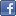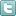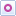EE Times-India > T&M

T&M# Employ correlation to measure phase difference

Posted: 30 Dec 2015Print Version

Keywords:oscilloscope  algorithm  PLL generated clocks  FPGA  Correlation

Performing mathematical operations on the signals can enhance the phase measurement. Techniques described in references 1, 2, and 3 are some examples of such operations. Although each method might be suitable for certain applications, the measurements suffer from several factors beyond the scope of this article. In addition, these techniques are mostly for sinusoidal signals. In applications such as measuring the phase performance of the various PLL generated clocks inside an FPGA, they are markedly inaccurate.

Correlation
A simple and accurate method is to correlate the signals. Correlation is a straight-forward mathematical operation. Numerous papers (Ref. 4) thoroughly explain its operation as well as its applications. A C# algorithm, developed by Analog Arts, is one implementation of this technique. Figure 3 shows the phase difference between two 200MHz sine waves obtained by this algorithm.Figure 3: Correlation lets you calculate phase difference between two signals.

A key advantage of correlation is its ability to find the phase difference between most other types of signals. Figure 4 shows the phase difference between a sine wave and a square wave. In addition, issues such as the DC content of the signals, noise, and triggering problems have insignificant effect on the result. For applications where the signal noise becomes a dominant factor, averaging can be used to reduce its effect.Figure 4: Phase difference between a sine wave and a square wave using correlation.

The accuracy, which can be obtained by this technique, is mainly limited by the relative accuracy of the period of the signals and the sampling rate of the oscilloscope. For a signal sampling rate of 100GHz and a relative frequency accuracy of .01 ppm, phase measurements of better than .5 degrees can be expected. Signal averaging can further enhance the accuracy of the measurements to .1 degree.

Correlation proves to be beneficial in real world applications where issues such as, ringing, reflection, and rise-time mismatch between the signals severely limit other phase measurement techniques.

References
1. G. Huang, L.R. Doolittle, J.W. Staples, R. Wilcox, J.M. Byrd, Signal Processing for High Precision Phase Measurements
2. Measuring relative phase between two waveforms using an oscilloscope. http://eeshop.unl.edu/pdf/OscilloscopeTutorial—PhaseMeasurement.pdf
3. Peter O'Shea, Phase Measurement
4. Correlation functions and their application for the analysis of MD results. http://people.virginia.edu/~lz2n/mse627/notes/Correlations.pdf

 Related Articles Editor's Choice
Comment on "Employ correlation to measure phase ..."

Top Ranked Articles

WebinarsVisit Asia Webinars to learn about the latest in technology and get practical design tips.

Search EE Times India
Services

Go to topConnect on FacebookFollow us on TwitterFollow us on Orkut
﻿